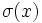# Strict characteristicity is strongly intersection-closed

Jump to: navigation, search
This article gives the statement, and possibly proof, of a subgroup property satisfying a subgroup metaproperty
View all subgroup metaproperty satisfactions | View all subgroup metaproperty dissatisfactions |Get help on looking up metaproperty (dis)satisfactions for subgroup properties
|
Property "Page" (as page type) with input value "{{{property}}}" contains invalid characters or is incomplete and therefore can cause unexpected results during a query or annotation process.
Property "Page" (as page type) with input value "{{{metaproperty}}}" contains invalid characters or is incomplete and therefore can cause unexpected results during a query or annotation process.

## Statement

### Verbal statement

An arbitrary (possibly empty) intersection of strictly characteristic subgroups of a group is strictly characteristic.

Note: The use of the word strongly is to allow the empty intersection as well. We can also say that normality is intersection-closed and also identity-true.

### Symbolic statement

Let$I$ be an indexing set and$H_i$ be a family of characteristic subgroups of$G$ indexed by$I$. Then, the intersection, over all$i$ in$I$, of the strictly characteristic subgroups$H_i$, is also a strictly characteristic subgroup of$G$.

## Definitions used

### Fully invariant subgroup

A subgroup$N$ of a group$G$ is said to be strictly characteristic, if given any surjective endomorphism$\sigma$ of$G$, we have$\sigma(N)$$N$.

### Strongly intersection-closed

A subgroup property is termed strongly intersection-closed if given any family of subgroups having the property, their intersection also has the property. Note that just saying that a subgroup property is intersection-closed simply means that given any nonempty family of subgroups with the property, the intersection also has the property.

Thus, the property of being strongly intersection-closed is the conjunction of the properties of being intersection-closed and identity-true, viz satisfied by the whole group as a subgroup of itself.

## Generalizations

The general result (of which this can be viewed as a special case) is that any invariance property is strongly intersection-closed.

Here, an invariance property is the property of being invariant with respect to a certain collection of functions on the whole group. For strictly characteristic subgroups, the collection of functions is the surjective endomorphisms.

## Proof

Let$H_i$ be a family of strictly characteristic subgroups of$G$ indexed by$I$. Suppose$H$ is the intersection. We need to show that$H$ is strictly characteristic in$G$. In other words, for any$x$ in$H$ and any surjective endomorphism$\sigma$ of$G$, we need to show that$\sigma(x)$ is also in$H$.

Now we know that since$x$ is in$H$,$x$ is also in each$H_i$. By the normality of$H_i$,$\sigma(x)$ is contained in$H_i$. Hence,$\sigma(x)$ is also contained in the intersection of all the$H_i$s.

This proof generalizes to the one for an arbitrary invariance property. The idea behind the generalization is that we in no way used any information about the nature and structure of endomorphisms -- they were a black box for us.